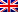#[Cybercamp 2015] [Crypto 11-19] Write Up

## Crypto 11

s=not(t)
s= a xor b
t= b xor c
a=1010

c = ?

This was some easy XOR calculation too :

s xor t = a xor (b xor b) xor c
s xor t = a xor c
s xor t = 1010 xor c
s xor t = c xor 1010

But since s = not(t)
s xor t = 1111

We then have

1111 = c xor 1010

So

c = 0101

## Crypto 13

2/4, 3/7, 5/12, 8/19, 13/30, 21/43
Find the next one.

Numerators are Fibonacci numbers.

Denominators are prime numbers. So the following number is

34/60

## Crypto 14

Find the next seed for the pseudo-random sequence :

0 1 3 6 10

First seed is +1, next one is +2 then +2, +4 so next one is

5

## Crypto 15

HOLA = 292
MUNDO = 387

What is the key hidden in GOTA AZUL, knowing that spaces are represented by zeros ?

We notice that 292 is the sum of ascii values of HOLA letters, same thing for 387 and MUNDO. So the key hidden in GOTA AZUL is :

2990316

## Crypto 17

We had to encrypt “EL RETO COMIENZA” with AES-128 cipher, using the key 1619701717150b6f630a0169170b0e0e. Goal is to find the matrix right after the first operation of the cypher.

Using the PHPAES class, we had all the ciphering in php code. We just had to set the initial variable, and break right after the first operation (addRoundKey).

Initialisation gave us the following matrix :

```E E C E
L T O N
O M Z
R&nbsp;&nbsp; I A```

S R   R U A E E P D L T E O   O[/code

So the seeked string was SUPERADO EL RETO and the flag was

## Crypto 19

p = 262944577762774866106056967998748809727
q = 272272036199178794499477884067503714423

n = 71592455595004021823287239861496066635645600363495430966317367141122572592521
phi(n) = 71592455595004021823287239861496066635110383749533477305711832289056320068372
e = 65537

public (n,e)
private (n,d)

We found d :
d = 26130148432679191937577716060957717227090656869994671363544669856023729740993

Which was the flag !